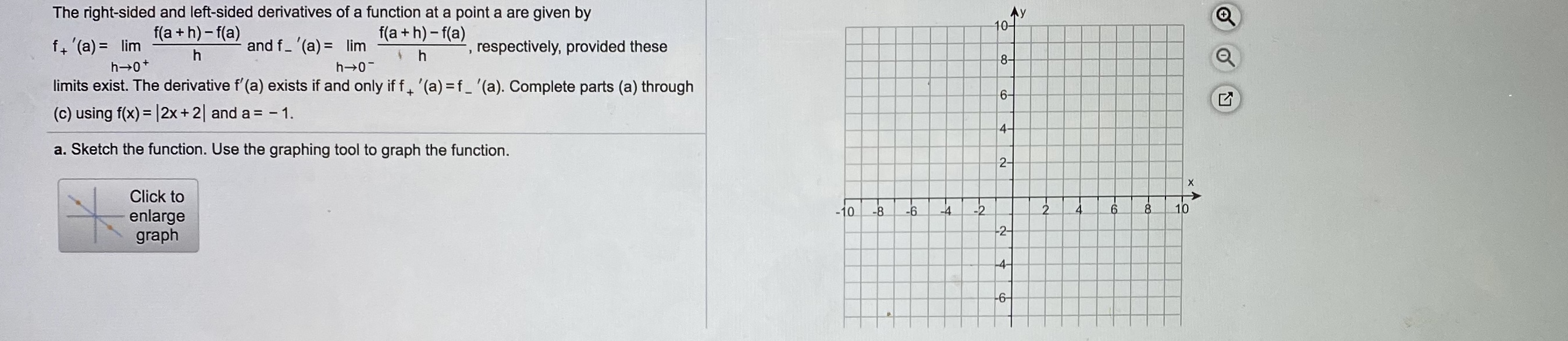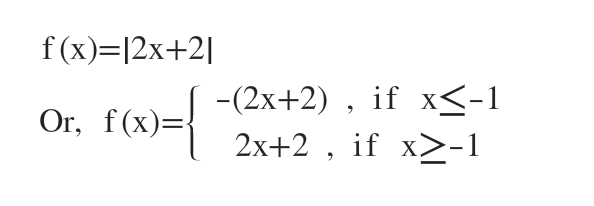# The right-sided and left-sided derivatives of a function at a point a are given byAy10-f(a + h) - f(a)f(a + h)- f(a)f, '(a) = limh 0+limits exist. The derivative f'(a) exists if and only if f. '(a) =f_ '(a). Complete parts (a) through(c) using f(x) = |2x+2| and a = - 1.and f- (a) = limrespectively, provided these8-h 0-6-%3D4-a. Sketch the function. Use the graphing tool to graph the function.2-Click to108.2.-24-4-10-8-6enlargegraph-2--4-6in

Question
1 views

these are notes from class, i was absenthelp_outlineImage TranscriptioncloseThe right-sided and left-sided derivatives of a function at a point a are given by Ay 10- f(a + h) - f(a) f(a + h)- f(a) f, '(a) = lim h 0+ limits exist. The derivative f'(a) exists if and only if f. '(a) =f_ '(a). Complete parts (a) through (c) using f(x) = |2x+2| and a = - 1. and f- (a) = lim respectively, provided these 8- h 0- 6- %3D 4- a. Sketch the function. Use the graphing tool to graph the function. 2- Click to 10 8. 2. -2 4 -4 -10 -8 -6 enlarge graph -2- -4 -6 in fullscreen
check_circle

Step 1

Given function is f(x)= |2x+2|

a) We have to graph the function.

2x+2=0

or, 2x=-2

or, x=-1

So at x=-1 there is vertex.

Step 2Using this piecewise function we make two tables.

 x -5 -4 -3 -2 -1 y 8 6 4 2 0

 x 0 1 2 3 4 y 2 4 6 8 10
...

### Want to see the full answer?

See Solution

#### Want to see this answer and more?

Solutions are written by subject experts who are available 24/7. Questions are typically answered within 1 hour.*

See Solution
*Response times may vary by subject and question.
Tagged in

### Calculus